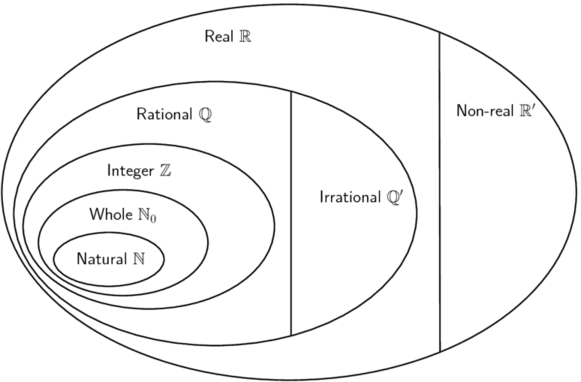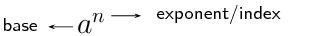Home Practice
For learners and parents For teachers and schools
Textbooks
Full catalogue
Pricing SupportLog in

We think you are located in United States. Is this correct?

# Chapter 1: Exponents and surds

## 1.1 Revision (EMBF2)

### The number system (EMBF3)

• Discuss the number system; explain the difference between real and non-real numbers.
• Encourage learners not to use calculators in this chapter.
• Common misconception: $$\pi$$ (irrational) ≈ $$\frac{22}{7}$$ (rational).
• Explain that the square root of a negative number is non-real.
• Discuss raising a negative number to even and odd powers.
• Explain that surds are a special notation or way of expressing rational exponents.
• Key strategy in manipulation of exponential expressions: express base in terms of its prime factors.
• Emphasize the principle of equivalence and using the additive inverse in the simplification of equations (and not “simply taking term to the other side”).
• Rationalising the denominators is a useful tool for working with special angles in Trigonometry.
• Learners should leave their final answers as mixed fractions.
• Answers should always be written with positive exponents.

The diagram below shows the structure of the number system:We use the following definitions:

• $$\mathbb{N}$$: natural numbers are $$\{1; \; 2; \; 3; \; \ldots\}$$

• $$\mathbb{N}_0$$: whole numbers are $$\{0; \; 1; \; 2; \; 3; \; \ldots\}$$

• $$\mathbb{Z}$$: integers are $$\{\ldots; \; -3; \; -2; \; -1; \; 0; \; 1; \; 2; \; 3; \; \ldots\}$$

• $$\mathbb{Q}$$: rational numbers are numbers which can be written as $$\frac{a}{b}$$ where $$a$$ and $$b$$ are integers and $$b\ne 0$$, or as a terminating or recurring decimal number.

Examples: $$-\frac{7}{2}; \; -\text{2,25}; \; 0; \; \sqrt{9}; \; \text{0,}\dot{8}; \; \frac{23}{1}$$

• $$\mathbb{Q}'$$: irrational numbers are numbers that cannot be written as a fraction with the numerator and denominator as integers. Irrational numbers also include decimal numbers that neither terminate nor recur.

Examples: $$\sqrt{3}; \; \sqrt{2}; \; \pi; \; \frac{1 + \sqrt{5}}{2}; \; \text{1,27548}\ldots$$

• $$\mathbb{R}$$: real numbers include all rational and irrational numbers.

• $$\mathbb{R}'$$: non-real numbers or imaginary numbers are numbers that are not real.

Examples: $$\sqrt{-25}; \; \sqrt{-1}; \; -\sqrt{-\frac{1}{16}}$$

temp text

## The number system

Textbook Exercise 1.1

Use the list of words below to describe each of the following numbers (in some cases multiple words will be applicable):

• Natural ($$\mathbb{N}$$)
• Whole ($$\mathbb{N}_0$$)
• Integer ($$\mathbb{Z}$$)
• Rational ($$\mathbb{Q}$$)
• Irrational ($$\mathbb{Q}'$$)
• Real ($$\mathbb{R}$$)
• Non-real ($$\mathbb{R}'$$)

$$\sqrt{7}$$

$$\mathbb{R}; \mathbb{Q}'$$

$$\text{0,01}$$

$$\mathbb{R}; \mathbb{Q}$$

$$16\frac{2}{5}$$

$$\mathbb{R}; \mathbb{Q}$$

$$\sqrt{6\frac{1}{4}}$$

$$\mathbb{R}; \mathbb{Q}$$

$$\text{0}$$

$$\mathbb{R}; \mathbb{Q}; \mathbb{Z}; \mathbb{N}_0$$

$$2\pi$$

$$\mathbb{R}' \mathbb{Q}'$$

$$-\text{5,3}\dot{8}$$

$$\mathbb{R}; \mathbb{Q}$$

$$\frac{1-\sqrt{2}}{2}$$

$$\mathbb{R}; \mathbb{Q}'$$

$$-\sqrt{-3}$$

$$\mathbb{R}'$$

$$(\pi)^2$$

$$\mathbb{R}; \mathbb{Q}'$$

$$-\frac{9}{11}$$

$$\mathbb{R}; \mathbb{Q}$$

$$\sqrt{-8}$$

$$\mathbb{R}; \mathbb{Q}; \mathbb{Z}$$

$$\frac{22}{7}$$

$$\mathbb{R}; \mathbb{Q}$$

$$\text{2,45897}\ldots$$

$$\mathbb{R}; \mathbb{Q}'$$

$$\text{0,}\overline{65}$$

$$\mathbb{R}; \mathbb{Q}$$

$$\sqrt{-32}$$

$$\mathbb{R}; \mathbb{Q}; \mathbb{Z}$$

### Laws of exponents (EMBF4)

We use exponential notation to show that a number or variable is multiplied by itself a certain number of times. The exponent, also called the index or power, indicates the number of times the multiplication is repeated.$a^n = a \times a \times a \times \ldots \times a \quad (n \text{ times}) \qquad \left(a \in \mathbb{R}, n \in \mathbb{N}\right)$

Examples:

1. $$2 \times 2 \times 2 \times 2 = 2^4$$
2. $$\text{0,71} \times \text{0,71} \times \text{0,71} = (\text{0,71})^3$$
3. $$(\text{501})^2 = \text{501} \times \text{501}$$
4. $$k^6 = k \times k \times k \times k \times k \times k$$

For $$x^2$$, we say $$x$$ is squared and for $$y^3$$, we say that $$y$$ is cubed. In the last example we have $$k^6$$; we say that $$k$$ is raised to the sixth power.

We also have the following definitions for exponents. It is important to remember that we always write the final answer with a positive exponent.

• $${a}^{0}=1$$ ($$a \ne 0$$ because $$0^0$$ is undefined)

• $${a}^{-n}=\frac{1}{{a}^{n}}$$ ($$a \ne 0$$ because $$\frac{1}{0}$$ is undefined)

Examples:

1. $$5^{-2} = \dfrac{1}{5^2} = \dfrac{1}{25}$$
2. $$(-36)^0 x = (1)x = x$$
3. $$\dfrac{7p^{-1}}{q^{3}t^{-2}} = \dfrac{7t^2}{pq^3}$$

We use the following laws for working with exponents:

• $${a}^{m} \times {a}^{n}={a}^{m+n}$$
• $$\frac{{a}^{m}}{{a}^{n}}={a}^{m-n}$$
• $${\left(ab\right)}^{n}={a}^{n}{b}^{n}$$
• $${\left(\frac{a}{b}\right)}^{n}=\frac{{a}^{n}}{{b}^{n}}$$
• $${\left({a}^{m}\right)}^{n}={a}^{mn}$$
where $$a > 0$$, $$b > 0$$ and $$m, n \in \mathbb{Z}$$.

## Worked example 1: Laws of exponents

Simplify the following:

1. $$5(m^{2t})^p \times 2(m^{3p})^t$$
2. $$\dfrac{8k^3x^2}{(xk)^2}$$
3. $$\dfrac{2^2 \times 3 \times 7^4}{(7 \times 2)^4}$$
4. $$3(3^b)^a$$
1. $$5(m^{2t})^p \times 2(m^{3p})^t = 10m^{2pt + 3pt} = 10m^{5pt}$$
2. $$\dfrac{8k^3x^2}{(xk)^2} = \dfrac{8k^3x^2}{x^2k^2} = 8k^{(3-2)}x^{(2-2)} = 8k^1x^0 = 8k$$
3. $$\dfrac{2^2 \times 3 \times 7^4}{(7 \times 2)^4} = \dfrac{2^2 \times 3 \times 7^4}{7^4 \times 2^4} = 2^{(2-4)} \times 3 \times 7^{(4-4)} = 2^{-2} \times 3 = \frac{3}{4}$$
4. $$3(3^b)^a = 3 \times 3^{ab} = 3^{ab + 1}$$
temp text

## Worked example 2: Laws of exponents

Simplify:$$\dfrac{3^m - 3^{m+1}}{4 \times 3^m - 3^m}$$

### Simplify to a form that can be factorised

$\dfrac{3^m - 3^{m+1}}{4 \times 3^m - 3^m} = \dfrac{3^m - (3^{m} \times 3)}{4 \times 3^m - 3^m}$

### Take out a common factor

\begin{align*} &= \dfrac{3^m(1 - 3)}{3^m(4 - 1)} \end{align*}

### Cancel the common factor and simplify

\begin{align*} &= \frac{1 - 3}{4 - 1} \\ &= - \frac{2}{3} \end{align*}
temp text

## Laws of exponents

Textbook Exercise 1.2

Simplify the following:

$$4 \times 4^{2a} \times 4^2 \times 4^a$$

\begin{align*} 4 \times 4^{2a} \times 4^2 \times 4^a &= 4^{1+2a+2+a} \\ &= 4^{3a+3} \end{align*}

$$\dfrac{3^2}{2^{-3}}$$

\begin{align*} \dfrac{3^2}{2^{-3}} &= 3^2 \times 2^3 \\ &= 9 \times 8 \\ &= 72 \end{align*}

$$(3p^5)^2$$

\begin{align*} (3p^5)^2 &= 3^2 \times p^{10} \\ &= 9 p^{10} \end{align*}

$$\dfrac{k^2k^{3x-4}}{k^x}$$

\begin{align*} \dfrac{k^2k^{3x-4}}{k^x} &= \dfrac{k^{3x-2}}{k^x} \\ &= k^{3x-2-(x)} \\ &= k^{2x-2} \end{align*}

$$(5^{z-1})^2+5^z$$

$(5^{z-1})^2+5^z = 5^{2z-2}+5^z$

$$(\frac{1}{4})^0$$

$\left ( \frac{1}{4} \right )^0 = 1$

$$(x^2)^5$$

$\left ( x^2 \right )^5 = x^{10}$

$$\left( \frac{a}{b} \right)^{-2}$$

\begin{align*} \left ( \frac{a}{b} \right )^{-2} &= \frac{a^{-2}}{b^{-2}} \\ &= \frac{b^2}{a^2} \end{align*}

$$(m+n)^{-1}$$

$\left ( m+n \right )^{-1} = \frac{1}{m+n}$

$$2(p^t)^s$$

$2\left ( p^t \right )^s = 2p^{ts}$

$$\dfrac{1}{\left(\frac{1}{a}\right)^{-1}}$$

$\dfrac{1}{\left ( \frac{1}{a} \right )^{-1}} = \dfrac{1}{a}$

$$\frac{k^{0}}{k^{-1}}$$

$\frac{k^{0}}{k^{-1}} = k$

$$\dfrac{-2}{-2^{-a}}$$

\begin{align*} \dfrac{-2}{-2^{-a}} &= 2 \times 2^a \\ &= 2^{a+1} \end{align*}

$$\dfrac{-h}{(-h)^{-3}}$$

\begin{align*} \frac{-h}{\left ( -h \right )^{-3}} &= -h\left ( -h \right )^3 \\ &= -h\left ( -h^3 \right ) \\ &= h^4 \end{align*}

$$\left( \dfrac{a^2b^3}{c^3d} \right)^2$$

$\left ( \frac{a^2b^3}{c^3d} \right )^2 = \frac{a^4b^6}{c^6d^2}$

$$10^{7}(7^{0}) \times 10^{-6}(-6)^{0}-6$$

\begin{align*} 10^{7}\left ( 7^{0} \right ) \times 10^{-6}\left ( -6 \right )^{0}-6 &= 10^7(1) \times 10^{-6}(1) - 6 \\ &= 10^1 - 6 \\ &= 4 \end{align*}

$$m^3n^2 \div nm^2 \times \frac{mn}{2}$$

\begin{align*} m^3n^2 \div nm^2 \times \frac{mn}{2} &= m^3n^2 \times \frac{1}{m^2n} \times \frac{mn}{2} \\ &= \frac{m^3n^2}{m^2n} \times \frac{mn}{2} \\ &= \frac{m^2n^2}{2} \end{align*}

$$(2^{-2}-5^{-1})^{-2}$$

\begin{align*} \left ( 2^{-2}-5^{-1} \right )^{-2} &= \left ( \frac{1}{4} - \frac{1}{5} \right )^{-2} \\ &= \left ( \frac{1}{20} \right )^{-2} \\ &= 20^2 \\ &= \text{400} \end{align*}

$$(y^2)^{-3} \div \left( \frac{x^2}{y^3} \right)^{-1}$$

\begin{align*} \left ( y^2 \right )^{-3} \div \left ( \frac{x^2}{y^3} \right )^{-1}\div \frac{y^{-2}}{x^{-2}} &= \frac{1}{y^6} \times \frac{x^2}{y^3} \times \frac{y^{2}}{x^{2}} \\ &= \frac{1}{y^7} \end{align*}

$$\dfrac{2^{c-5}}{2^{c-8}}$$

\begin{align*} \dfrac{2^{c-5}}{2^{c-8}} &= 2^{(c-5)-(c-8)} \\ &= 2^{c-5-c+8} \\ &= 2^{3} \\ &= 8 \end{align*}

$$\dfrac{2^{9a} \times 4^{6a} \times 2^2}{8^{5a}}$$

\begin{align*} \dfrac{2^{9a} \times 4^{6a} \times 2^2}{8^{5a}} &= \frac{2^{9a} \times 2^{12a} \times 2^2}{2^{15a}} \\ &= \frac{2^{9a+12a+2}}{2^{15a}} \\ &= 2^{21a+2-15a} \\ &= 2^{6a+2} \end{align*}

$$\dfrac{20t^5p^{10}}{10t^4p^9}$$

\begin{align*} \dfrac{20t^5p^{10}}{10t^4p^9} &= 2t^{5-4}p^{10-9} \\ &= 2pt \end{align*}

$$\left( \dfrac{9q^{-2s}}{q^{-3s}y^{-4a-1}} \right)^2$$

\begin{align*} \left( \dfrac{9q^{-2s}}{q^{-3s}y^{-4a-1}} \right)^2 &= \frac{\left( 9q^{-2s} \right)^2}{\left( q^{-3s}y^{-4a-1} \right)^2} \\ &= \frac{ 81q^{-4s} }{ q^{-6s}y^{-8a-2} } \\ &= \frac{ 81q^{6s} }{ q^{4s}y^{-(8a+2)} } \\ &= 81q^{2s} y^{8a+2} \end{align*}